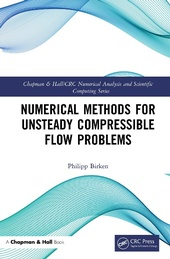# Numerical Methods for Unsteady Compressible Flow Problems

Chapman & Hall/CRC (Verlag)
• erschienen am 4. Juli 2021
• |
• 246 Seiten

 E-Book | PDF ohne DRM | Systemvoraussetzungen
978-1-000-40352-7 (ISBN)

Numerical Methods for Unsteady Compressible Flow Problems is written to give both mathematicians and engineers an overview of the state of the art in the field, as well as of new developments. The focus is on methods for the compressible Navier-Stokes equations, the solutions of which can exhibit shocks, boundary layers and turbulence. The idea of the text is to explain the important ideas to the reader, while giving enough detail and pointers to literature to facilitate implementation of methods and application of concepts.

The book covers high order methods in space, such as Discontinuous Galerkin methods, and high order methods in time, in particular implicit ones. A large part of the text is reserved to discuss iterative methods for the arising large nonlinear and linear equation systems. Ample space is given to both state-of-the-art multigrid and preconditioned Newton-Krylov schemes.

Features

• Applications to aerospace, high-speed vehicles, heat transfer, and more besides

• Suitable as a textbook for graduate-level courses in CFD, or as a reference for practitioners in the field

 Reihe: Sprache: Englisch Verlagsort: Milton | Großbritannien Verlagsgruppe: Taylor & Francis Ltd Zielgruppe: Für höhere Schule und Studium Illustrationen: 54 schwarz-weiße Abbildungen, 54 schwarz-weiße Zeichnungen Dateigröße: 10,30 MB Schlagworte: ISBN-13: 978-1-000-40352-7 (9781000403527)weitere Ausgaben werden ermittelt

Philipp Birken is an associate professor for numerical analysis and scientific computing at the Centre for the Mathematical Sciences at Lund University, Sweden. He received his PhD in mathematics in 2005 from the University of Kassel, Germany, where he also received his habilitation in 2012. He was a PostDoc and a consulting assistant professor at the Institute for Computational & Mathematical Engineering at Stanford University, USA. His work is in numerical methods for compressible CFD and Fluid-Structure-Interaction.

Preface. 1. Introduction. 1.1. The method of lines. 1.2. Hardware. 1.3. Notation. 1.4. Outline. 2. The Governing Equation. 2.1. The Navier-Stokes Equations. 2.2. Nondimensionalization. 2.3. Source terms. 2.4. Simplifications of the Navier-Stokes equations. 2.5. The Euler Equations. 2.6. Solution theory. 2.7. Boundary layers. 2.8. Boundary layers. 2.9. Laminar and turbulent flows. 3. The Space discretization. 3.1. Structured and unstructured Grids. 3.2. Finite Volume Methods. 3.3. The Line Integrals and Numerical Flux Functions. 3.4 Convergence theory for finite volume methods. 3.5. Source Terms. 3.6. Finite volume methods of higher order. 3.7. Discontinuous Galerkin methods. 3.8. Convergence theory for DG methods. 3.9. Boundary Conditions. 3.10. Spatial Adaptation. 4. Time Integration Schemes. 4.1. Order of convergence and order of consistency. 4.2 Stability. 4.3. Stiff problems. 4.4. Backward Differentiation formulas. 4.5. Runge-Kutta methods. 4.6. Rosenbrock-type methods. 4.7. Adaptive time step size selection. 4.8. Operator Splittings. 4.9. Alternatives to the method of lines. 4.10. Parallelization in time. 5. Solving equation systems. 5.1. The nonlinear systems. 5.2. The linear systems. 5.3. Rate of convergence and error. 5.4. Termination criteria. 5.5. Fixed Point methods. 5.6. Multigrid methods. 5.7. Newton's method. 5.8. Krylov subspace methods. 5.9. Jacobian Free Newton-Krylov methods. 5.10. Comparison of GMRES and BiCGSTAB. 5.11. Comparison of variants of Newton's method. 6. Preconditioning linear systems. 6.1. Preconditioning for JFNK schemes. 6.2. Specific preconditioners. 6.3. Preconditioning in parallel. 6.4. Sequences of linear systems. 6.5. Discretization for the preconditioner. 7. The final schemes. 7.1. DIRK scheme. 7.2. Rosenbrock scheme. 7.3. Parallelization. 7.4. Efficiency of Finite Volume schemes. 7.5. Efficiency of Discontinuous Galerkin schemes. 8. Thermal Fluid Structure Interaction. 8.1. Gas Quenching. 8.2. The mathematical model. 8.3. Space discretization. 8.4. Coupled time integration. 8.5. Dirichlet-Neumann iteration. 8.6. Alternative solvers. 8.7. Numerical Results. A. Test problems. A.1. Shu-Vortex. A.2. Supersonic Flow around a cylinder. A.3. Wind Turbine. A.4. Vortex shedding behind a sphere. B. Coefficients of time integration methods. Bibliography. Index.

Schweitzer Klassifikation
• Mathematik
•Analysis
• Mathematik
•Angewandte Mathematik
•Numerische Mathematik
• Physik / Astronomie
•Mechanik
•Strömungsmechanik
Thema Klassifikation
DNB DDC Sachgruppen
• Naturwissenschaften
•Physik
Dewey Decimal Classfication (DDC)
• Science
•Physics
•Fluid mechanics
BIC Classifikation
• Mathematics & science
•Mathematics
•Applied mathematics
• Mathematics & science
•Mathematics
•Calculus & mathematical analysis
•Numerical analysis
• Mathematics & science
•Physics
•Classical mechanics
•Fluid mechanics
BISAC Classifikation
• Mathematics
•AppliedDateiformat: PDF
Kopierschutz: ohne DRM (Digital Rights Management)

Systemvoraussetzungen:

Computer (Windows; MacOS X; Linux): Verwenden Sie zum Lesen die kostenlose Software Adobe Reader, Adobe Digital Editions oder einen anderen PDF-Viewer Ihrer Wahl (siehe E-Book Hilfe).

Tablet/Smartphone (Android; iOS): Installieren Sie die kostenlose App Adobe Digital Editions oder eine andere Lese-App für E-Books (siehe E-Book Hilfe).

E-Book-Reader: Bookeen, Kobo, Pocketbook, Sony, Tolino u.v.a.m. (nur bedingt: Kindle)

Das Dateiformat PDF zeigt auf jeder Hardware eine Buchseite stets identisch an. Daher ist eine PDF auch für ein komplexes Layout geeignet, wie es bei Lehr- und Fachbüchern verwendet wird (Bilder, Tabellen, Spalten, Fußnoten). Bei kleinen Displays von E-Readern oder Smartphones sind PDF leider eher nervig, weil zu viel Scrollen notwendig ist. Ein Kopierschutz bzw. Digital Rights Management wird bei diesem E-Book nicht eingesetzt.

Weitere Informationen finden Sie in unserer E-Book Hilfe.